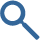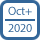# With 96 SPSS Statistics guides, use Laerd Statistics as your definitive SPSS Statistics resource.

We have a wide range of SPSS Statistics guides to help you analyse your data, from the more straightforward to the more advanced. These include statistical tests to help you determine if there are differences between groups, predict scores, identify associations, perform data reduction, and test for assumptions. We also show you how to create graphs and charts, as well as get started and set up your data in SPSS Statistics. A list of the SPSS Statistics guides in our enhanced content is provided below. You can see the new guides we're adding to the site here.

•• Statistical Tests

 Differences between groups Independent-samples t-test Paired-samples t-test One-way ANOVA Repeated measures ANOVA Two-way ANOVA Factorial (three-way) ANOVA Within-within-subjects ANOVA Three-way repeated measures ANOVA Three-way mixed ANOVA Mixed ANOVA Hotelling's T2 One-way MANOVA Two-way MANOVA One-way MANCOVA One-way ANCOVA Two-way ANCOVA Mann-Whitney U test Wilcoxon signed-rank test Kruskal-Wallis H test Jonckheere-Terpstra test Friedman test McNemar's test Cochran's Q test Sign test Test of two proportions Chi-square test of homogeneity (2 x C) Chi-square test of homogeneity (R x 2) One sample One-sample t-test Chi-square goodness-of-fit Survival analysis Kaplan-Meier Reliability Cronbach's Alpha Cohen's kappa Fleiss' kappa Weighted kappa Kendall's W Predicting scores Linear regression Multiple regression Hierarchical multiple regression Logistic regression Ordinal regression Associations Pearson's correlation Point-biserial correlation Pearson's partial correlation Spearman's correlation Kendall's τb (tau-b) Goodman and Kruskal's γ (gamma) Somers' d Mantel-Haenszel test of trend Cochran-Armitage test of trend Chi-square test for association (2x2) Chi-square test of independence (RxC) Fisher's exact test (2x2) for independence Relative risk (2 x 2) Odds ratio (2 x 2) Goodman and Kruskal's λ (lambda) Loglinear analysis Data reduction Principal components analysis Assumptions Testing for normality Transforming data Moderation Dichotomous moderator analysis Graphs and charts Bar chart Clustered bar chart Simple error bar chart Simple 3-D bar chart Simple line graph Multiple line graph Simple area chart Stacked area chart Simple scatterplot Grouped scatterplot Scatterplot matrix Simple dot plot Simple 3-D scatterplot Simple histogram Stacked histogram Frequency polygon Boxplot Clustered boxplot 1-D boxplot Pie chart Population pyramid Getting to know SPSS Creating a new file Opening a file Data setup in SPSS Reverse coding in SPSS Selecting cases in SPSS Splitting a file Weighting cases Mean centring continuous variables Creating dummy variables Calculating a total score Calculating a mean score Creating an ID variable Recoding a continuous to an ordinal variable Recoding a continuous to a dichotomous variable Calculating a z-score (standard score)

We are adding guides all the time, and have a schedule of new SPSS Statistics guides that we will be adding in October 2020 and beyond. We also maintain a "wishlist" from our users, which forms the basis of our schedule. Therefore, if there is a particular guide you want that you don't see below, please contact us. We can let you know when we will be able to add a suitable guide to the site.

•• Cox regression, multinomial logistic regression, one-way repeated measures MANOVA, intraclass correlation coefficient (ICC), Poisson regression, negative binomial regression, and introductions to linear mixed models (LMM) and generalised estimating equations (GEE)